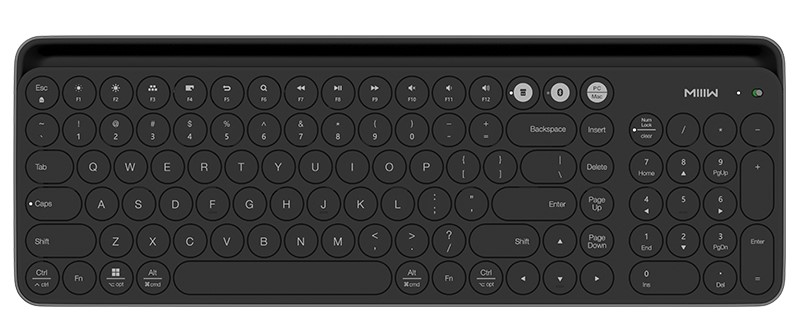0%

618还是没能忍住，看上一款米家的无线键盘，感觉用来码字，写✍BUG😂还是蛮不错的。2019

__Merry Christmas !__ 祝大家圣诞快乐！ [ 冒个泡，证明还活着 (￣▽￣)" ]$$S_1=\frac{m_霸}{M+m_霸+m_神}L$$

$$S_2=\frac{m_神}{M+m_霸+m_神}L$$

$$m_神=\frac{m_霸L-\left ( M+m_霸 \right )\left ( S_1-S_2 \right )}{L+S_1-S_2}$$

#### MTProxy 简介

MTProxy 是专为 Telegram 打造的代理工具，也只能代理 Telegram 。

#### MTProxy 安装

##### 安装依赖项

• Debian/Ubuntu:

• CentOS/RHEL:

• Git获取MTProxy源代码: